# IB DP Chemistry: SL复习笔记2.1.6 Energy Levels & Sublevels

### Electron Energy Levels

#### Shells

• The arrangement of electrons in an atom is called the electronic configuration
• Electrons are arranged around the nucleus in principal energy levels or principal quantum shells
• Principal quantum numbers (n) are used to number the energy levels or quantum shells
• The lower the principal quantum number, the closer the shell is to the nucleus
• The higher the principal quantum number, the lesser the energy of the shell
• Each principal quantum number has a fixed number of electrons it can hold
• n = 1 : up to 2 electrons
• n = 2 : up to 8 electrons
• n = 3 : up to 18 electrons
• n = 4 : up to 32 electrons
• There is a pattern here - the mathematical relationship between the number of electrons and the principal energy level is 2n
• So for example, in the third shell n = 3 and the number of electrons is 2 x (32 ) = 18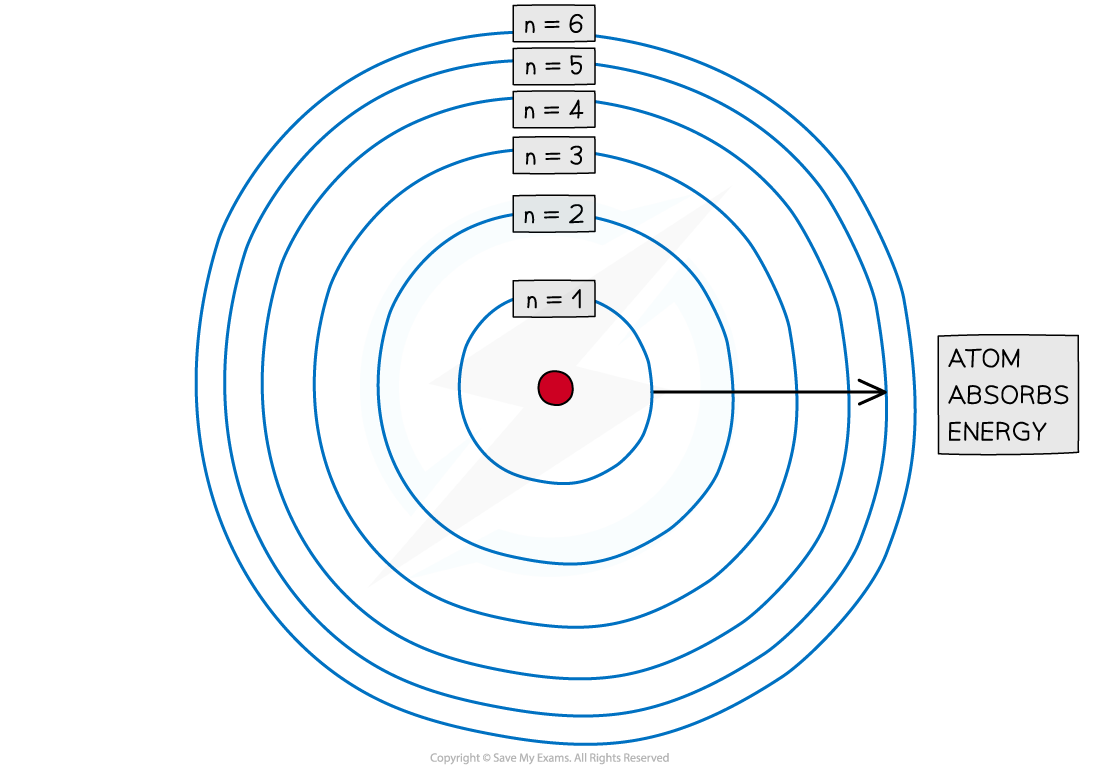Electrons are arranged in principal quantum shells, which are numbered by principal quantum numbers

#### Subshells

• The principal quantum shells are split into subshells which are given the letters sp and d
• Elements with more than 57 electrons also have an f subshell
• The energy of the electrons in the subshells increases in the order s < p < d
• The order of subshells overlap for the higher principal quantum shells as seen in the diagram below: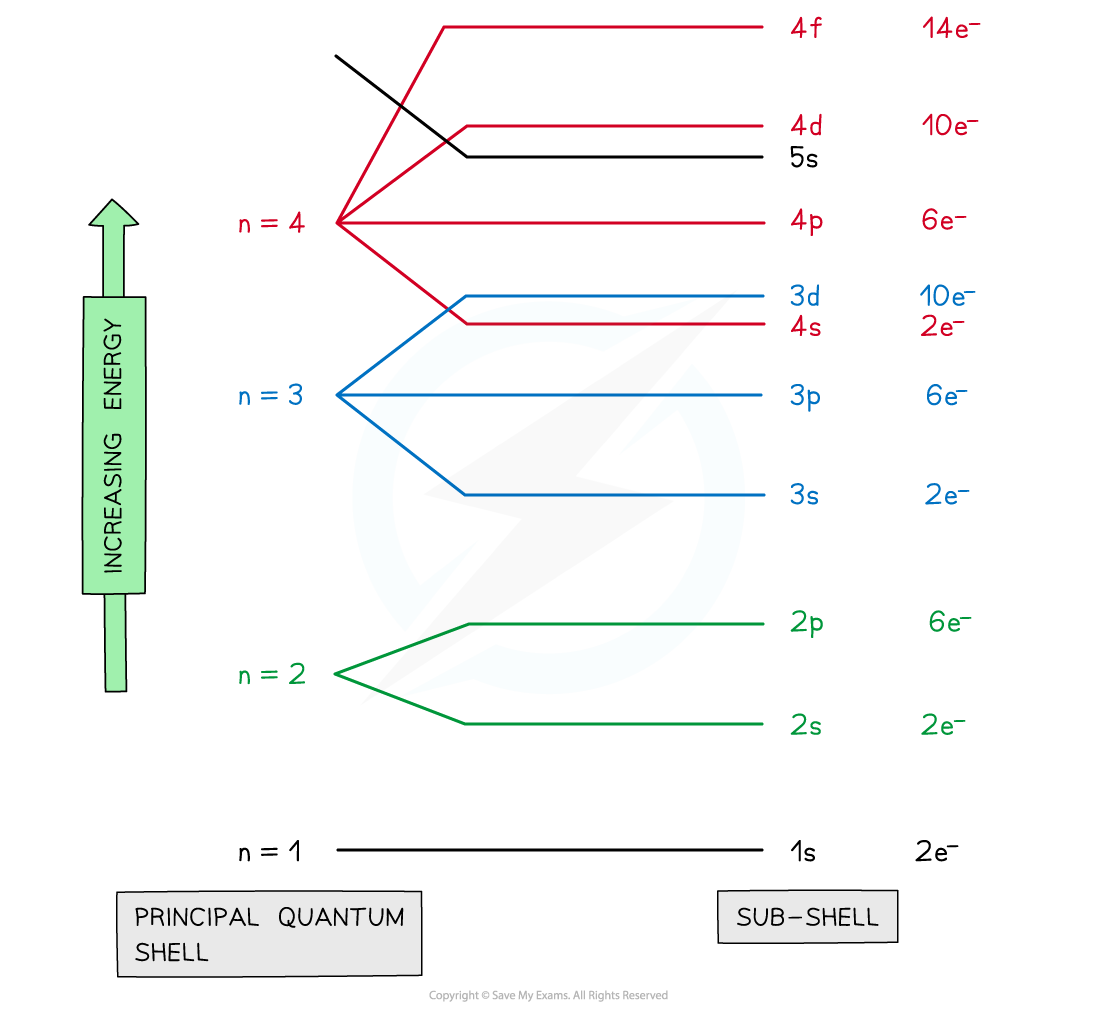Electrons are arranged in principal quantum shells, which are numbered by principal quantum numbers

#### Orbitals

• The subshells contain one or more atomic orbitals
• Orbitals exist at specific energy levels and electrons can only be found at these specific levels, not in between
• Each atomic orbital can be occupied by a maximum of two electrons
• The orbitals have specific 3D shapes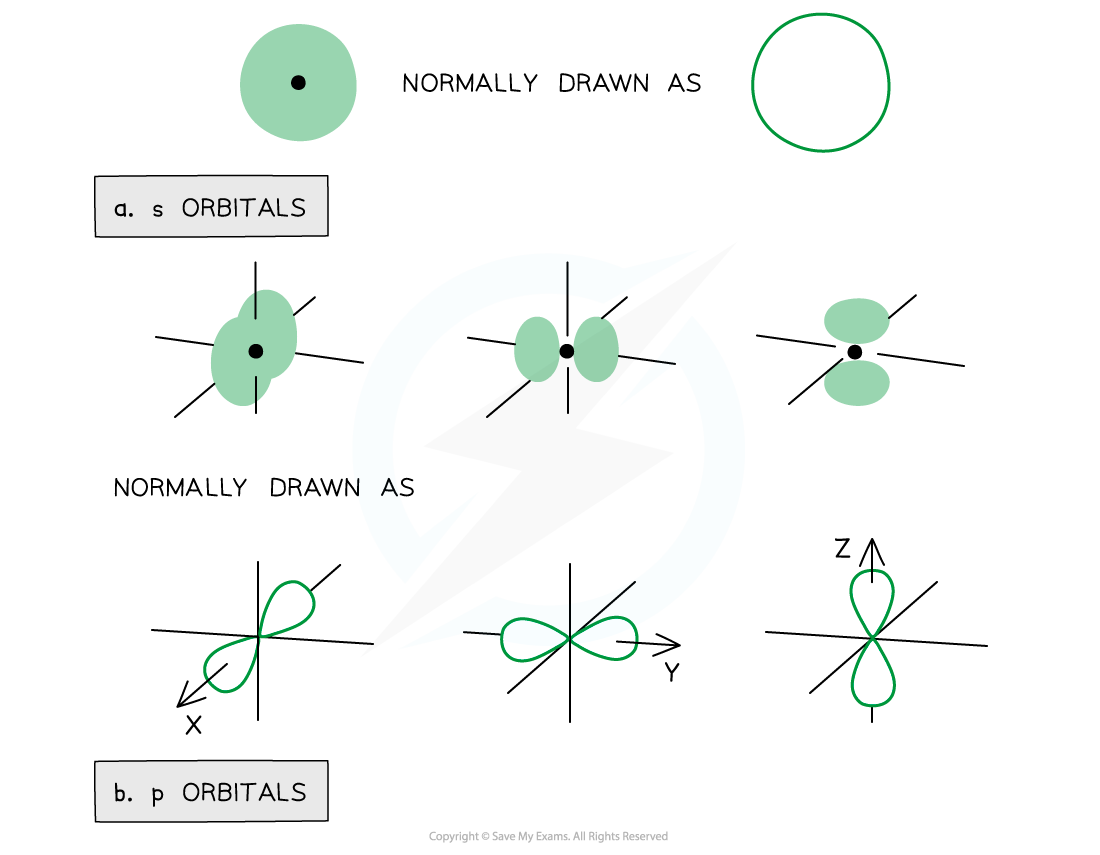Representation of orbitals (the dot represents the nucleus of the atom) showing spherical s orbitals (a), p orbitals containing ‘lobes’ along the x, y and z axis

• Note that the shape of the d orbitals is not required for IB Chemistry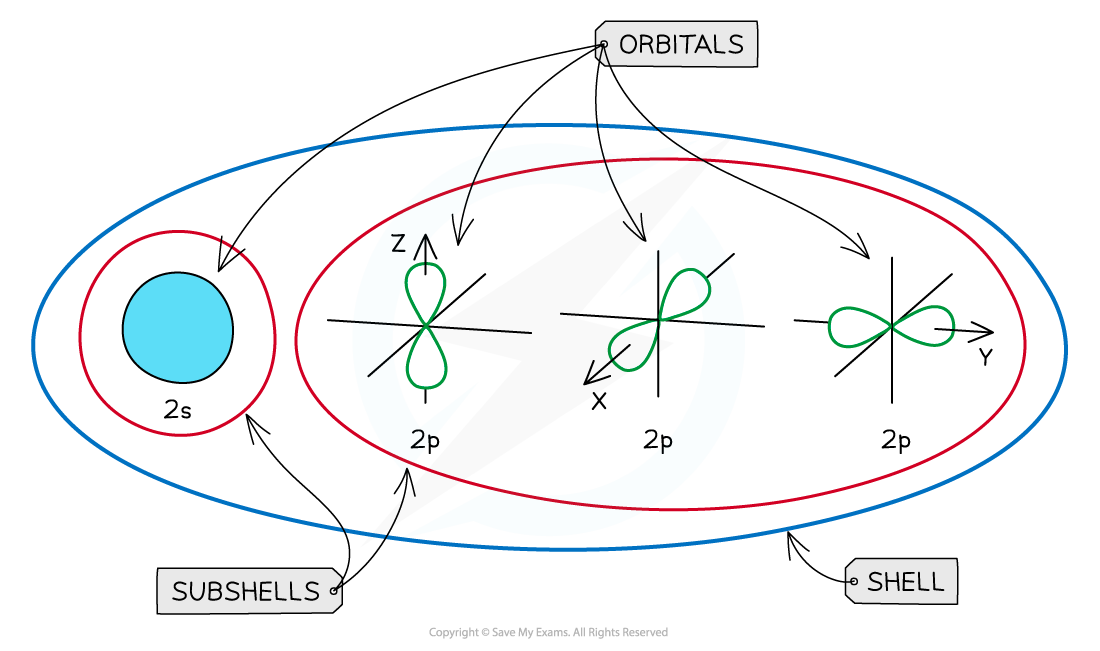An overview of the shells, subshells and orbitals in an atom

#### Ground state

• The ground state is the most stable electronic configuration of an atom which has the lowest amount of energy
• This is achieved by filling the subshells of energy with the lowest energy first (1s) - this is called the Aufbau Principle
• The order of the subshells in terms of increasing energy does not follow a regular pattern at n= 3 and higher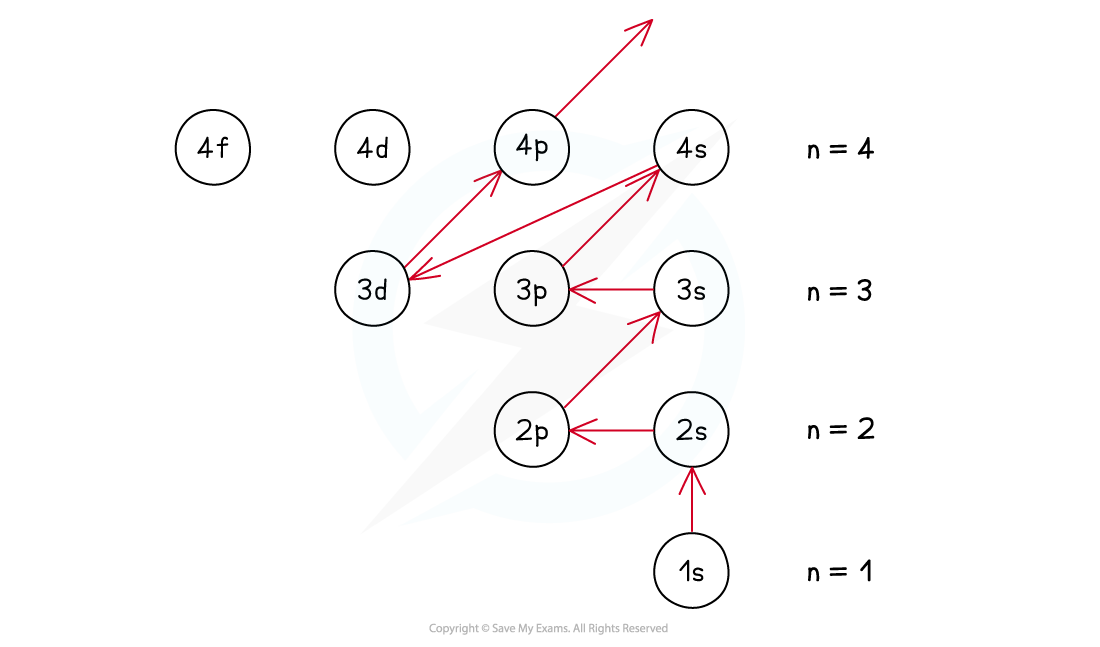The Aufbau Principle - following the arrows gives you the filling order

### Sublevels & Energy

• The principal quantum shells increase in energy with increasing principal quantum number
• Eg. n = 4 is higher in energy than n = 2
• The subshells increase in energy as follows: s ＜ p ＜ d ＜ f
• The only exception to these rules is the 3d orbital which has slightly higher energy than the 4s orbital, so the 4s orbital is filled before the 3d orbital
• All the orbitals in the same subshell have the same energy and are said to be degenerate
• Eg. px, py and pz are all equal in energy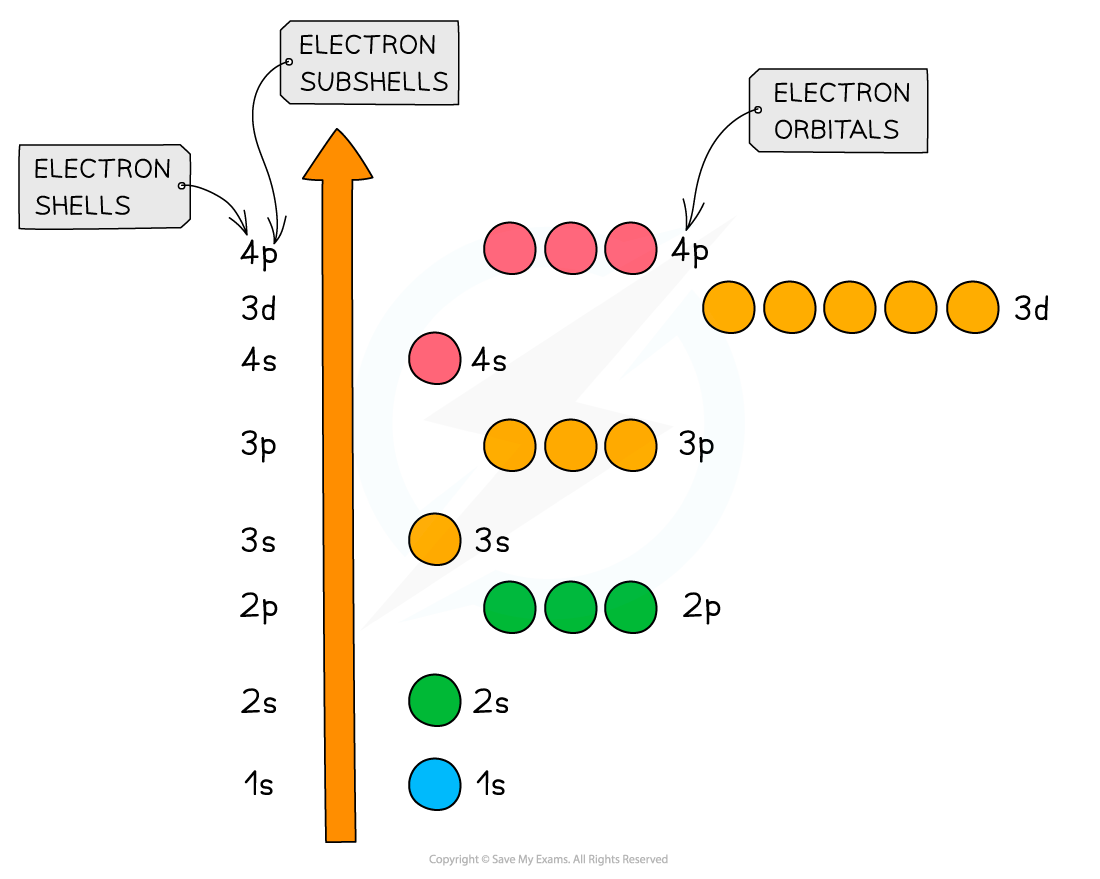Relative energies of the shells and subshells